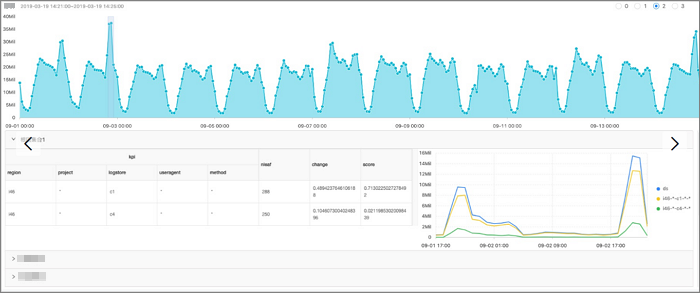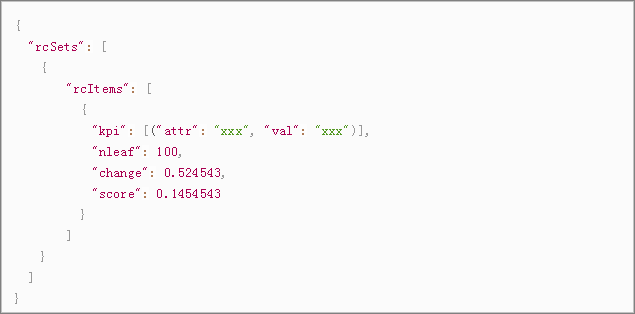Log Service provides powerful alerting and analysis capabilities that help you quickly analyze and locate the subdimensions of abnormal metrics. When a time series metric is abnormal, you can use the root cause analysis function to quickly analyze the dimension attributes that result in the abnormal metric.

rca_kpi_search

Function format:
select rca_kpi_search(varchar_array, name_array, real, forecast, level)
The following table lists the parameters of the function.
Parameter Description Value
varchar_array The dimensions. Array. Example: array[col1, col2, col3].
name_array The dimension attributes. Array. Example: array['col1', 'col2', 'col3'].
real The actual value of each dimension specified by varchar_array. Double type. Valid values: all real numbers.
forecast The predicted value of each dimension specified by varchar_array. Double type. Valid values: all real numbers.
level The number of dimensions corresponding to the output root cause sets. A value of 0 indicates that all root cause sets that are found are returned. Long type. Valid values: [0, number of analyzed dimensions]. The number of analyzed dimensions is the number of elements in the array specified by the varchar_array parameter.
Example:
• The query statement is as follows.

The query statement uses a subquery to obtain the actual value and predicted value of each fine-grained attribute, and then calls the rca_kpi_search function to analyze the root cause of the exception.

* not Status:200 |
select rca_kpi_search(
array[ ProjectName, LogStore, UserAgent, Method ],
array[ 'ProjectName', 'LogStore', 'UserAgent', 'Method' ], real, forecast, 1)
from (
select ProjectName, LogStore, UserAgent, Method,
sum(case when time < 1552436040 then real else 0 end) * 1.0 / sum(case when time < 1552436040
then 1 else 0 end) as forecast,
sum(case when time >=1552436040 then real else 0 end) *1.0 / sum(case when time >= 1552436040
then 1 else 0 end) as real
from (
select __time__ - __time__ % 60 as time, ProjectName, LogStore, UserAgent, Method, COUNT(*) as real
from log GROUP by time, ProjectName, LogStore, UserAgent, Method )
GROUP BY ProjectName, LogStore, UserAgent, Method limit 100000000)
• The following figure shows the output result.The following figure shows the structure of the output result.The following table describes the display items.
Display item Description
rcSets The root cause sets. Each value is an array.
rcItems The root cause set.
kpi The KPI in the root cause set, which is an array. Each value in the array is in JSON format. attr indicates a dimension, and val indicates an attribute in the dimension.
nleaf The number of leaves that the current KPI covers in the raw data.
Note A leaf is a log for the finest-grained attributes.
change The ratio of changes in the leaves covered by the current KPI to the total changes at the same time point.
score The abnormality score of the current KPI. Valid values: [0, 1].
The output result is in JSON format as follows:
{
"rcSets": [
{
"rcItems": [
{
"kpi": [
{
"attr": "country",
"val": "*"
},
{
"attr": "province",
"val": "*"
},
{
"attr": "provider",
"val": "*"
},
{
"attr": "domain",
"val": "example.com"
},
{
"attr": "method",
"val": "*"
}
],
"nleaf": 119,
"change": 0.3180687806279939,
"score": 0.14436007709620113
}
]
}
]
}